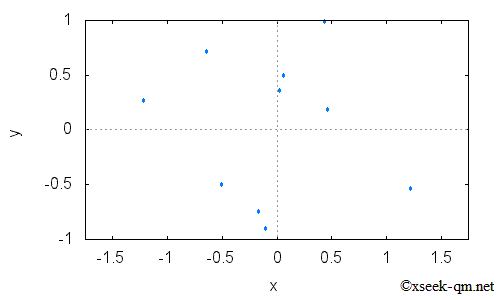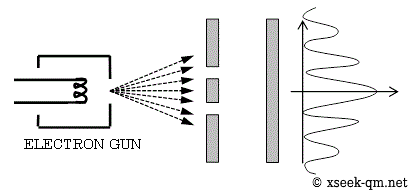# The double-slit experiment of quantum mechanics

2014/1/26
Published 2002/2/14

I would like to serve as a guide to the entrance of quantum theory on this website, catering to those unfamiliar with physics. I hope to pique your interest in this mysterious and fascinating field.

## The double-slit experiment

To begin, I would like to introduce the double-slit experiment, a hallmark of quantum mechanics.An electron travels toward a wall with two slits. The electron can either collide with the wall or pass through one of the slits. If it passes through a slit, it will continue on to strike a second wall. If many electrons are allowed to pass through the slits, some will collide with the second wall, as depicted in the following figure.If we increase the number of electrons that hit the wall, the figure depicted above transforms into the following figure.A stripe pattern appears as the number of electrons impacting the wall increases. This pattern reflects the distribution of electrons, which can be observed in the figure below."What is the source of this stripe pattern? Electrons possess wave-like properties, and when waves pass through two slits, they can either constructively or destructively interfere with each other. This phenomenon is called interference, and it is responsible for the creation of the stripe pattern observed in the double-slit experiment. This result is enigmatic, as individual electrons pass through the slits one at a time, yet the collective behavior of the electrons exhibits wave-like interference patterns. How is it that we are able to observe this interference pattern, despite the fact that it arises from the passage of discrete particles?"

## Why is the pattern of stripes resulting from interference formed?

The wave of the electron is expressed by the following mathematical function.

Ψ (x)

The wave function of an electron is described by the following function. This function is referred to as the wave function and is represented as "psi of x." The variable x represents the position of the electron, and the wave function is a function of complex numbers. It describes the state of the electron.

In the double-slit experiment, the electron exhibits two possible paths - one through the right slit, and the other through the left. We can represent the wave function of the wave passing through the right slit as ΨR (x ) and the wave function of the wave passing through the left slit as ΨL (x ). The superposition of these wave functions is achieved by adding ΨR (x ) and ΨL (x ).

Ψ (x) = ΨR (x) + ΨL (x)

The interference arises from this superposition. It is referred to as the superposition principle. The probability of observing an electron at a particular position, P(x), is the square of the absolute value of the wave function.

P (x ) = |Ψ (x ) |2

Why is the probability of observation of electrons equal to the square of the absolute value of the wave function?

The physicist Feynman, in his 1965 textbook "The Feynman Lectures on Physics".

One might still like to ask: "How does it work? What is the machinery behind the law?"

No one has found any machinery behind the law. No one can "explain" any more than we have just "explained." No one will give you any deeper representation of the situation. We have no ideas about a more basic mechanism from which these results can be deduced.

Therefore, we are unaware of the mechanism. I would like to reference a quote from Einstein, dating back to 1951 from the textbook "Gauge Theories in Particle Physics".

All the fifty years of conscious brooding have brought me no closer to the answer to the question, "What are light quanta?" Of course today every rascal thinks he knows the answer, but he is deluding himself.

I would like to delve into the enigmatic nature of quantum theory with you on this website.

Related Articles: